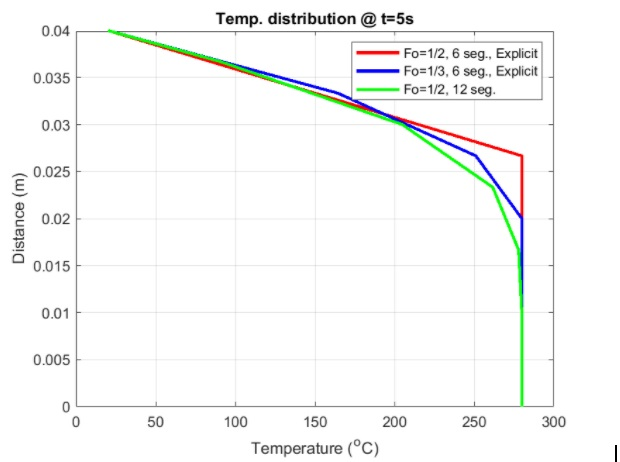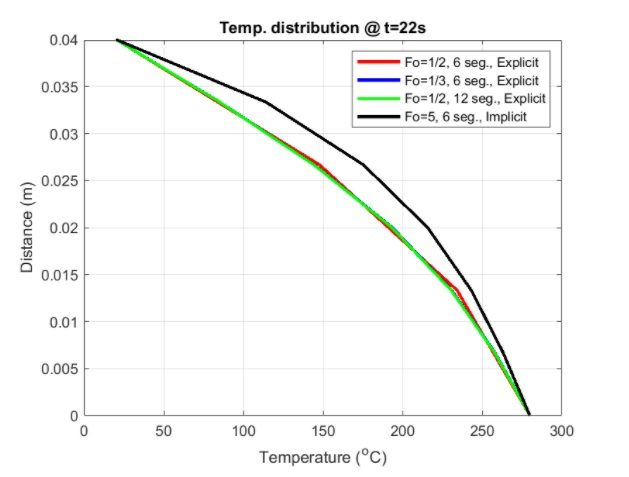• Assistance with numerical analysis assignment on deriving the Fourier number equation
• Numerical Analysis

## Assistance with numerical analysis assignment on deriving the Fourier number equation

The 1D equation can be written as:

${\rho C_p \frac {\partial T}{\partial t}= k \frac {\partial^2 T}{\partial x^2}}$

If we discretize this numerical analysis assignment equation by using central differences for the diffusion term (right-hand side) and an explicit method for the time-derivative, it will help us get the following equation:

${\rho C_p \frac{T^{t+1}_t-T^t_t}{\Delta t}= \frac{k}{\Delta x^2}(T^t_{j-1}-2T^t_j+T^t_{j+1})}$

That can be rearranged to obtain

${T^{t+1}_j-T^t_j=Fo(T^t_{j-1}-2T^t_j+T^t_{j+1})}$

Where Fo is the Fourier number:

${Fo= \frac{k \Delta t}{\rho C_p \Delta x^2}}$

Therefore, Fo is a function of the time step and spatial resolution. The explicit method was implemented in For all the cases a), b), c), and d) by using different combinations of Fo and the number of segments:

The results for C were extended up to 200s as the timestep obtained from the conditions given is 22.2

The model under the conditions of C – Explicit is not stable as the time step is too large.

### Help with evaluating the accuracy of the Fourier number in numerical analysis homework

The results are also presented in the following plots. Figure 1 shows the profiles at 5s (or the closest to 5s). The most accurate results for this numerical analysis homework is obtained for D, which helped in identifying the best spatial resolution as 12 segments are considered. It was conducted with the same number of segments, and therefore it is shown that the most accurate result is obtained for Fo=1/3 that corresponds to a smaller timestep. This means that the accuracy increases with higher resolution and smaller timesteps (that actually means a better resolution in time). Finally, it does not converge since the timestep is too large to be used with an explicit method.Figure 1: Profile of Temperature at t=5s.

For an implicit discretization in time, the equation comes:

${T^{t+1}_j-T^t_j= Fo(T^{t+1}_{j-1}-2T^{t+1}_j+T^{t+1}_{j+1})}$

This equation can be rearranged as follows:

${a_pT_p+a_eT_e+a_wT_w=b_j}$

Where ${T_p=T^{t+1}_j,T_e=T^{t+1}_{j+1},T_w=T^{t+1}_{j-1},}$ and ${b_j=T^t_p.}$ Therefore:

ap=2*Fo

ae=-Fo

aw=-Fo

### Thomas algorithm application by online numerical analysis tutors

The above system can be solved using the Thomas algorithm in more complex cases. For this simple test, the online numerical analysis tutor solved the system using the backslash division.

Figure 2 shows the results for a, b,d, and c-implicit at 22s – note that the time step obtained for 22s and therefore, it is not possible to present the results as 15s as required in the assignment). The first important conclusion is that unlike the explicit method with Fo=5, it actually converges. This means that implicit methods allow much larger time steps, which is important for more complex simulations that are computationally heavier. The second important conclusion is that the result obtained with the implicit method differs substantially from all the other methods and, more importantly, is more accurate as the implicit methods are generally better at representing the diffusion.

## Numerical AnalysisFigure 1: Profile of Temperature at t=22s.